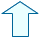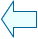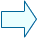THE AREA OF A POLYGON Section: Geometry

1. THE AREA OF A POLYGON
In order to work out the area of a regular polygon we need to know the following:
• the perimeter of a polygon is the length of all its sides. As the shape in the window is a hexagon the perimeter is equal to 6·side.
• the apothem is the line perpendicular to one of the sides of the polygon and joins this side to the centre of the shape.
1.- In the following window point R is found in the middle of one of the sides. Move point T to check that the apothem has the property of being the shortest segment
 Use the move_triangle control button to find out how many triangles equal to ODE there are in the shape.

3.- Notice that in this example the area of triangle ODE is:

area of triangle = (side·apothem)/2

as the base = the side of the polygon and the height = the apothem.

4.- Note that to work out the area of a polygon we need to find the area of ODE and then multiply it by 6. Therefore the complete area is:

area of polygon =6·(side·apothem)/2=(6·side·apothem)/2=
=(perimeter·apothem)/2Agustín Muñoz NúñezSpanish Ministry of Education. Year 2001